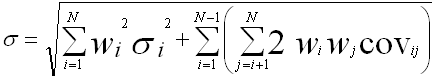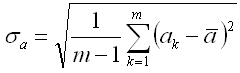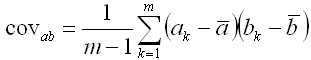Standard Deviation of Returns

Standard deviation of returns is a measure of volatility or risk.  The larger the return standard deviation, the larger the variations you can expect to see in returns.  Fund Manager can report this figure for any of these objects:  investment, symbol, asset type, investment goal, sector, investment type, or sub-portfolio.

#### Calculation Details:

When calculating the standard deviation of returns the simple monthly returns over the specified comparison period are calculated for every investment in the calculation object.  The simple monthly return is:

Return = (End_price + Dist_per_share - Start_price) / Start_price

If the comparison period is 5 years, there are 60 monthly returns.  The standard deviation of returns for the calculation object uses the formula:The objects standard deviation is a combination of the value weight and return standard deviation of each investment in the object.  It is also a function of the covariance in returns between each investment in the object.

N is the total number of investments in whatever object is being calculated (single investment, symbol, asset type, investment goal, sector, investment type, or sub-portfolio).  In the case where the calculation object is a single investment, N is 1.  In the case of a sub-portfolio with 10 investments, N will be 10, etc...

wi is the weight of that investment in the overall object being calculated.  The weight is determined by the ending value.

σi is the standard deviation of returns for that investment.  In the case where the comparison period is 5 years, there are 60 monthly returns calculated for each investment.  This is the standard deviation of those 60 returns for the i investment, using the regular standard deviation formula:covij is the covariance between the returns of investment i and investment j, using the regular covariance formula:where m is the number of return samples (60 for a 5 year comparison period), and a and b are the investment returns/averages for each investment.

When calculating returns your current interpolation range preferences are used.  If sufficient pricing data is not available the standard deviation will not be reported.

Fund Manager can also report an annualized standard deviation of returns.  This is simply the standard deviation of monthly returns times the square root of 12.

Fund Manager can output the data points used in this calculation to a log file.  By default the logging feature is turned off.  To turn it on, create a new registry string value at:

HKEY_CURRENT_USER/Software/Beiley Software/Fund Manager/CurrentVersion/techanal

named stddevretlog and set it to a value of 1.  The log file is named stddevret_log.txt, and will be located in your users AppData/Roaming/Fund Manager folder (usually a hidden folder).  This log file is deleted upon exiting Fund Manager.

## Reach out

#### Find us at the office

Exel- Frazzitta street no. 61, 44925 Kingston, Norfolk Island

#### Give us a ring

Jovanny Boeding
+23 274 947 142
Mon - Fri, 10:00-21:00